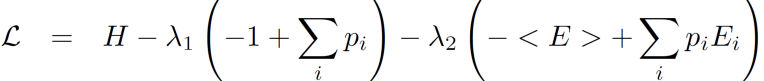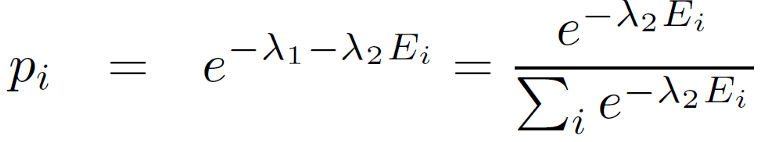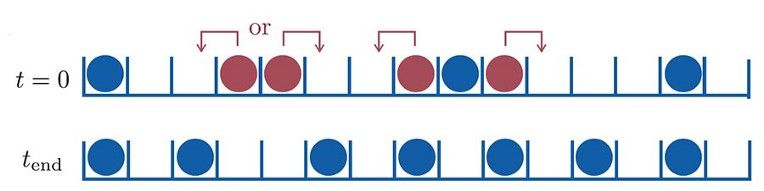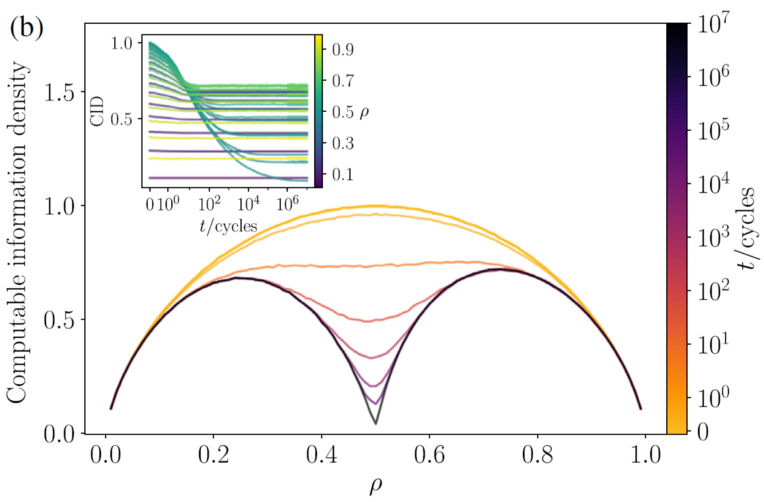# A first principles understanding of entropy as the measure information, and the foundation of statistical physicsSkanda Vivek

a year ago | 7 min read

Most of us first learn of entropy in the context of physics — whether it be the second law of thermodynamics or statistical mechanics. There is good reason for it, the concept of entropy was first introduced by Boltzmann in 1870 and later modified by Gibbs in 1902.

However, I believe the most intuitive understanding of entropy lies in information theory. In fact as you will see, statistical mechanics arises as a special consequence of maximal entropy subject to a constraint.

### Entropy in Information Theory

In 1948, Claude Shannon came up with a remarkable measure of information. This measure was not new, and he in fact recognizes this in his paper.

“The form of H will be recognized as that of entropy as defined in certain formulations of statistical mechanics where p_i is the probability of a system being in cell i of its phase space. H is then, for example, the H in Boltzmann’s famous H theorem.”

What was remarkable, was the relevance of the same measure to information theory, a feat for which Shannon is often referred to as the “father of information theory.” So how does this serve as a measure of information?

Take the example of a coin toss. There’s 2 choices — head (1) or tail (0), essentially a “bit” of information. (Fun fact: Shannon’s article was one of the first to use the concept of bits of information.) Each of the 2 choices has a probability of 1/2, giving it a Shannon Entropy of 1 bit — i.e. you need 1 bit to encode this information.

Now consider you have 4 options: 11, 00, 10, 01. If you go through the math, you find H=2, meaning you need 2 bits to encode the 4 possibilities given above. Now let’s go to why entropy is *the* measure of uncertainty, and by token, the measure of information.

If you have 2 possibilities, one having probability p, the other has probability q=1-p. Now if both possibilities are equally likely, the entropy turns out to be a maximum of 1 — which means you need 1 bit to encode the information (i.e. the coin toss example.)

Whereas if one possibility has probability of 1 (i.e. it always occurs), then the entropy turns out to be 0. This means there is no uncertainty — we 100% know the outcome; as in a weighted coin or a loaded dice. This brings us to a practical definition of entropy — a measure of certainty in the outcome of a process.

But why this measure and why not something else? As Shannon mentions, only H of this form satisfies 3 key laws:

1. H should be continuous in probabilities
2. With the increase in number of possible outcomes, there should be an increase in H. There is more uncertainty with more possible events
3. If a choice be broken down into successive choices, the original H should be the weighted sum of individual values of H.

Shannon then goes on to say:

This theorem, and the assumptions required for its proof, are in no way necessary for the present theory. It is given chiefly to lend a certain plausibility to some of our later definitions. The real justification of these definitions, however, will reside in their implications.

Meaning — the proof is in the pudding! The value of Shannon’s Entropy doesn’t lie in its proof of being the ultimate measure of information, but rather in its usefulness. After all, entropy has proved its value and continues to do so in statistical inference.

# Entropy in physics

In physics however, entropy plays a much more central role, and forms the basics of statistical mechanics. While entropy was coined in 1870, and later modified in 1902 by Gibbs, it was not viewed as essential to statistical mechanics, until Shannon’s famous paper. The connections between information theory and statistical mechanics were famously illustrated by Jaynes in 1957. According to Jaynes:

“(previously)…the identification of entropy was made only at the end, by comparison of the resulting equations with the laws of phenomenological thermodynamics. Now, however we can take entropy as our starting concept, and the fact that a probability distribution maximizes the entropy subject to certain constraints becomes the essential fact which justifies the use of that distribution for inference.”

He goes on to say:

In freeing the theory from its apparent dependence on physical hypotheses of the above type, we make it possible to see statistical mechanics in a more general light”

These are quite bold statements. Before, entropy was viewed as a side note and thermodynamics at the center of statistical mechanics. But Jaynes argues that entropy is more generally applicable, and thermodynamics is a special case of entropy maximization!

Lets break it down. At the heart of statistical mechanics is the Boltzmann distribution, which gives the probability that particles in a system have a certain energy as:

But remember Jaynes statement that probability in statistical mechanics maximizes entropy. However, we learnt from Shannon’s example applied to coins that a uniform distribution where every outcome is equally probable, maximizes entropy.

From where did the exponential distribution come from? For that, we need to remember the second part — maximizing entropy, *subject to certain constraints*. What then are these constraints?

The two constraints are the mean energy is fixed and all probabilities sum up to 1 (normalization). The second condition is easy to understand, for any system in equilibrium. The first condition reflects that energy of the system has a constant mean, which is proportional to the absolute temperature.

In order to solve the distribution that maximizes entropy subject to the 2 constraints above, you can use commonly used method of constrained optimization using Lagrange multipliers.Lagrangian function for maximization of entropy subject to the above constraints | Skanda Vivek

Inserting the Shannon entropy as H, you find the stationary points of the above Lagrangian function, which gives the required probability distribution as below, which is an inverse exponential, and the same form as the Boltzmann distribution!Maximal entropy distribution subject to the constraints of fixed mean energy and normalization conditions | Skanda Vivek

This distribution is the same as the Boltzmann distribution, replacing the constant in front of the energy. Thus, the starting point of statistical mechanics — the probability distribution, arises as a consequence of entropy maximization, albeit a special case.

An important technical note: The derived max entropy probability distribution represents the “Canonical Ensemble” in statistical mechanics, where the system is in thermal equilibrium with an infinite reservoir at fixed temperature, with which the system can exchange energy, and has a fixed mean energy <E>.

A more general distribution called the “Grand Canonical Ensemble” is obtained when the system can exchange both energy and particles with a large reservoir. Importantly, the constraints are constant mean energy <E>, as well as constant average particle number <N>.

The grand canonical ensemble probability is analogous to the canonical, with the additional dependence on number, N in the exponent. Thanks to

Mirco Milletarì Ph.D. for pointing this out!

### Information Entropy Reveals Hidden Order Out-of-Equilibrium

In going through seminal work, I’ve shown how statistical mechanics is a special case of entropy maximization. But keep in mind all of this is in the case of thermodynamic equilibrium — where there are no net energy flows, and the system variables do not change in time.

However, our world is filled with systems that are out of equilibrium. Examples range from amorphous materials, to biology, and even financial markets. In fact this is quite a deep unsolved problem.

For a system in equilibrium, by definition its macroscopic properties do not change in time. But systems such as financial markets do change in time, and are inherently out of equilibrium.

However, applications of equilibrium concepts to non-equilibrium systems can yield important information. Many physicists, as I have described in another article are of the view that non-equilibrium systems have some hidden features that have yet to be discovered, not as yielding as their equilibrium counterparts.

One quest is the search for hidden order in non-equilibrium systems. In recent work, a group of physicists showed that image file compression size can be used as a proxy for entropy in non-equilibrium systems.ID conserved lattice gas model | S. Martiniani, P. M. Chaikin, D. Levine, Phys. Rev. X 9, 011031, 2019. Thumbnail image credit: Bernd Luz, CC BY-SA 3.0.)

Using the common Lempel-Ziv 77 compression algorithm, they computed the ratio of reduced image file size after compression to file size before compression (Computable Information Density, or CID ) and used the ratio as a proxy for entropy.

They found that this CID reliable captured certain non-equilibrium phase transitions like the ID conserved lattice gas model shown above. Their CID captured the phase transition occurring at critical density=0.5, as seen from the divergence at long times.ID conserved lattice gas model CID Phase Transition | S. Martiniani, P. M. Chaikin, D. Levine, Phys. Rev. X 9, 011031, 2019

In summary, entropy is a concept with wide ranging applications in information theory and physics. Although entropy originated from statistical mechanics, within physics, it is more generally applicable and better understood from the perspective of information theory.

Over the years, through seminal work, we have learnt that statistical mechanics and thermodynamics are special consequences of entropy maximization. In fact, recent new research shows that information content as measured from file compression can provide new insights in non-equilibrium physics.

Simply put, the maximum entropy distribution is the ‘best guess’, based on partial information. When nothing is known, a uniform distribution is the best guess.

One can think of all physical laws, if not all laws as special consequences of maximum entropy. Paraphrasing Jaynes, a theory makes definite predictions of an experiment only if it leads to sharp distributions — which are the maximum entropy distributions corresponding to those theoretical assumptions.

UpvoteCreated by

Skanda Vivek

Senior Data Scientist in NLP. Creator of https://www.answerchatai.com/Post

Upvote

Downvote

Comment

Bookmark

Share

Related Articles